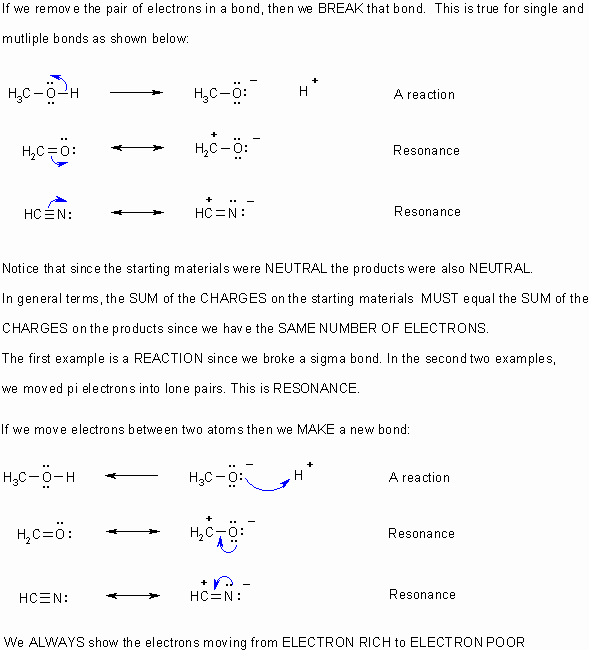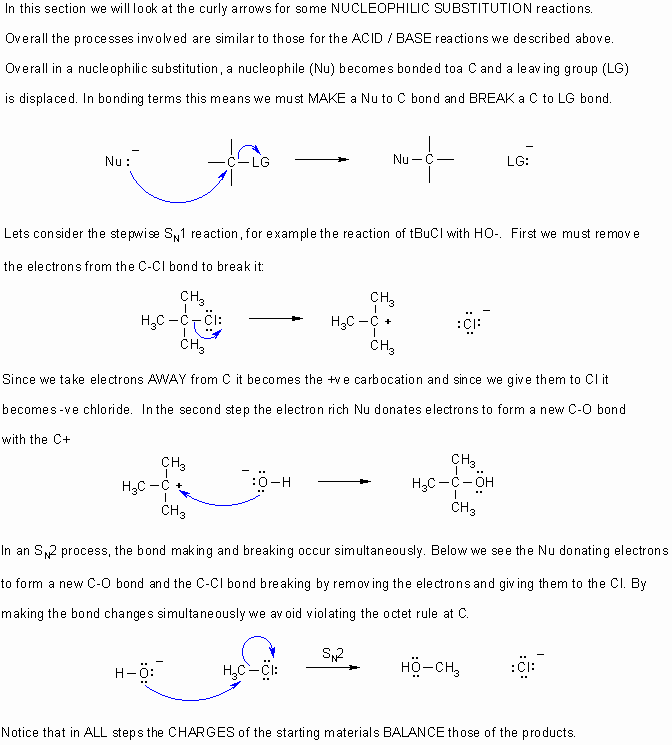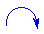# 1.6.1. Curved Arrow Notation

$$\newcommand{\vecs}{\overset { \rightharpoonup} {\mathbf{#1}} }$$ $$\newcommand{\vecd}{\overset{-\!-\!\rightharpoonup}{\vphantom{a}\smash {#1}}}$$$$\newcommand{\id}{\mathrm{id}}$$ $$\newcommand{\Span}{\mathrm{span}}$$ $$\newcommand{\kernel}{\mathrm{null}\,}$$ $$\newcommand{\range}{\mathrm{range}\,}$$ $$\newcommand{\RealPart}{\mathrm{Re}}$$ $$\newcommand{\ImaginaryPart}{\mathrm{Im}}$$ $$\newcommand{\Argument}{\mathrm{Arg}}$$ $$\newcommand{\norm}{\| #1 \|}$$ $$\newcommand{\inner}{\langle #1, #2 \rangle}$$ $$\newcommand{\Span}{\mathrm{span}}$$ $$\newcommand{\id}{\mathrm{id}}$$ $$\newcommand{\Span}{\mathrm{span}}$$ $$\newcommand{\kernel}{\mathrm{null}\,}$$ $$\newcommand{\range}{\mathrm{range}\,}$$ $$\newcommand{\RealPart}{\mathrm{Re}}$$ $$\newcommand{\ImaginaryPart}{\mathrm{Im}}$$ $$\newcommand{\Argument}{\mathrm{Arg}}$$ $$\newcommand{\norm}{\| #1 \|}$$ $$\newcommand{\inner}{\langle #1, #2 \rangle}$$ $$\newcommand{\Span}{\mathrm{span}}$$$$\newcommand{\AA}{\unicode[.8,0]{x212B}}$$

Objective

After completing this section, you should be able to use curved (curly) arrows, in conjunction with a chemical equation, to show the movement of electron pairs in a simple polar reaction, such as electrophilic addition.

##### Key Terms

Make certain that you can define, and use in context, the key terms below.

• electrophilic
• nucleophilic

## Pushing Electrons and Curly Arrows

Understanding the location of electrons and being able to draw the curly arrows that depict the mechanisms by which the reactions occur is one of the most critical tools for learning organic chemistry since they allow you to understand what controls reactions, and how reactions proceed.

Before you can do this you need to understand that a bond is due to a pair of electrons between atoms.

When asked to draw a MECHANISM, curly arrows should be used to show ALL the BONDING changes that occur.

A few simple lessons

## Lesson 1Lesson 2Lesson 3Lesson 4## Curly Arrow Summary• Curly arrowsflow from electron rich to electron poor.
• Therefore they start from lone pairs or bonds.
• The charges in any particular step should always be balanced.
• Remember to obey the rules of valence (eg. octet rule for C,N,O,F etc.)
• If electrons are taken out of a bond, then that bond is broken.
• If electrons are placed between two atoms then it implies a bond is being made.

## Exercises

Slide Presentations

Web Pages

Videos

Tutorial

Practice Problems

1.6.1. Curved Arrow Notation is shared under a CC BY-NC-SA 4.0 license and was authored, remixed, and/or curated by LibreTexts.